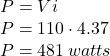## A coil with an inductance of 2.3 H and a resistance of 14 Ω is suddenly connected to an ideal battery with ε = 100 V. At 0.13 s after the co

Question

A coil with an inductance of 2.3 H and a resistance of 14 Ω is suddenly connected to an ideal battery with ε = 100 V. At 0.13 s after the connection is made, what is the rate at which (a) energy is being stored in the magnetic field, (b) thermal energy is appearing in the resistance, and (c) energy is being delivered by the battery?

in progress 0
6 months 2021-07-19T05:02:28+00:00 1 Answers 0 views 0

1. Given Information:

Resistance = R = 14 Ω

Inductance = L = 2.3 H

voltage = V = 100 V

time = t = 0.13 s

Required Information:

(a) energy is being stored in the magnetic field

(b) thermal energy is appearing in the resistance

(c) energy is being delivered by the battery?

(a) energy is being stored in the magnetic field ≈ 219 watts

(b) thermal energy is appearing in the resistance ≈ 267 watts

(c) energy is being delivered by the battery ≈ 481 watts

Explanation:

The energy stored in the inductor is given by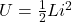The rate at which the energy is being stored in the inductor is given by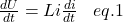The current through the RL circuit is given by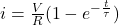Where τ is the the time constant and is given by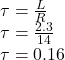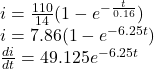Therefore, eq. 1 becomes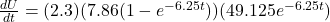At t = 0.13 seconds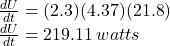(b) thermal energy is appearing in the resistance

The thermal energy is given by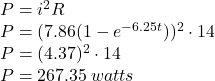(c) energy is being delivered by the battery?

The energy delivered by battery is